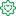Announcements
Product Release Webinar: Qlik Insider airing December 6! REGISTER TODAY!Former Employee

## The Only Function

There is a little known function in QlikView that hardly anyone uses and that doesn’t do very much, but still has a tremendous impact on many of the calculations made in QlikView.

It is the Only() function.

It returns the value of a parameter – but only if there is just one possible value. Hence, if you have a one-to-one relationship between the chart dimension and the parameter, the Only() function returns the only possible value back. But if there are several values, it returns NULL.The Only() function is an aggregation function, which means that it uses many records as input and returns one value only. The Sum() and Count() functions are examples of other aggregation functions. Aggregations are used whenever you have a group of records and need to show only one value representing all records.

When you think about it, QlikView uses aggregations in virtually all calculations: The expression in a chart, in a sort expression, in a text box, in an advanced search and in a calculated label are all aggregations and cannot be calculated without involving an aggregation function.

But what if the user enters an expression that lacks an explicit aggregation function? What does QlikView do then? For example, if the sort expression is set to “Date”? Or if there is an advanced search for customers using the expression “=Product='Shoe' ” (the intent is to find customers that have bought this product)?

This is where the Only() function affects the calculation without the user knowing it; if there is no explicit aggregation function in the expression, QlikView uses the Only() function implicitly. Hence, in the above cases, “Only(Date)” is used as sort expression and “=Only(Product)='Shoe' ” is used as search criterion.

Sometimes the new expression returns a result that the user does not expect. Both the above examples will work fine for cases when there is only one possible value of Date or Product, but neither of them will work for cases when there is more than one value.

Therefore, when you write expressions you should always ask yourself which aggregation you want to use, or: Which value do you want to use if there are several values? If the answer is that you want to use NULL to represent several values, then you indeed want to use the Only() function and you can leave the expression as it is.

But if you do not know the answer, then you should probably think again. For numbers, you probably want to use Sum(), Avg() or Min() instead and for strings you may want to use Only() or MinString(). For debugging you can always use something like, “Concat(distinct <Field>, ',')” and analyze the result.

But you should not leave your expression without an aggregation function.

HIC

Further reading related to this topic:

It’s all Aggregations

Use Aggregation Functions!